Courses

# Test: First Law Of Thermodynamics

## 20 Questions MCQ Test Chemistry Class 11 | Test: First Law Of Thermodynamics

Description
This mock test of Test: First Law Of Thermodynamics for Class 11 helps you for every Class 11 entrance exam. This contains 20 Multiple Choice Questions for Class 11 Test: First Law Of Thermodynamics (mcq) to study with solutions a complete question bank. The solved questions answers in this Test: First Law Of Thermodynamics quiz give you a good mix of easy questions and tough questions. Class 11 students definitely take this Test: First Law Of Thermodynamics exercise for a better result in the exam. You can find other Test: First Law Of Thermodynamics extra questions, long questions & short questions for Class 11 on EduRev as well by searching above.
QUESTION: 1

### Direction (Q. Nos. 1-10) This section contains 10 multiple choice questions. Each question has four choices (a), (b), (c) and (d), out of which ONLY ONE option is correct. Q. A gas is cooled and loses 65 J of heat. The gas contracts as it cools and work done on the system equal to 22 J is exchanged with the surroundings. Thus, q, W and ΔE (change in internal energy) are (in joules)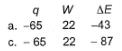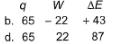Solution:

The IUPAC convention is as follow:-
For expansion or work done by the system on surrounding, W = negative
For compression or work done on system by surrounding, W = positive
Heat absorbed by the system is positive and heat released by the system is negative.
So, we have q = -65 J and W = 22 J
From 1st law of thermodynamics,
∆U = q+W
On putting values, we get ∆U = -43 J
So, q = -65 J, W = 22 J and ∆U = -43 J

QUESTION: 2

### Which of the following is true for a steady flow system?

Solution:

The amount of mass or energy entering a control volume does not have to be equal to the amount of mass or energy leaving during an unsteady-flow process.It is mostly converted to internal energy as shown by a rise in the fluid temperature.

QUESTION: 3

### One kg of carbon produces __________ kg of carbon dioxide.

Solution:

2CO + O2 ------------> 2CO2
Atomic wt of C =12 kg or gms, Atomic wt of O = 16 kg or gms
2(12 + 16) kg of CO + 32 kg of O2 -----------> 2(12+32) kg of CO2
56 kg of CO + 32 kg of O2 -------> 88 kg of CO2
For, 1kg of CO + 32/56 kg of O2 ---------> 88/56 kg of CO2
Therefore, 1kg of CO requires 32/56 or 4/7 kg of oxygen to produce 88/56kg or 11/7 kg of CO2

QUESTION: 4

A gas absorbs 200 J of heat and expands by 500 cm3 against a constant pressure of 2 x 105 Nm-2. Change in internal energy is

Solution:

The expression for the change in internal energy is given by,
ΔU=q+w
q = heat absorbed = 200 J
w = work done = −P x V
=−2×10⁵ × 500×10−6
= −100 N - m
ΔU=200−100J= 100 J
Hence, the correct option is C.

QUESTION: 5

The pressure-volume work for an ideal gas can be calculated using the expression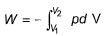This type of work can also be calculated using the area under the curve within the specified limits. When an ideal gas is compressed, (I) reversibly or (II) irreversibly, then

Solution:

Work done is the area under the P−V curve. It can be seen in the curve above that the area under the P−V curve for irreversible compression of the gas is more than the area under the curve for reversible compression.
Thus, work done for irreversible compression is more than that for reversible compression.

QUESTION: 6

In the following case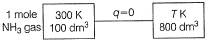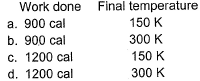Solution:

As q = 0, we have adiabatic process.
V1 = 100L and V2 = 800L
T1 = 300K and T2 = not given
For NH3, γ = 4/3
Applying TVγ = constant
(300)(100)4/3-1 = (T)(800)4/3-1
T = 300/2 = 150K
W= nR(T2-T1)/γ-1
= 1×8.314×150/(4/3-1)
= 3714 J
= 900 cal

QUESTION: 7

Assuming that water vapour is an ideal gas, internal energy change (ΔE)when 1 mole of water is vaporised at 1 bar pressure and 100°C will be

(Given, molar enthalpy of vaporisation of water at 1 bar and 373 K = 41 kJ mol-1)

[IIT JEE 2007]

Solution: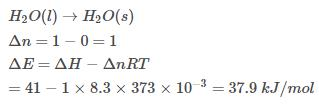QUESTION: 8

1.0 mole of a monoatomic ideal gas is expanded from state I to state II at 300 K.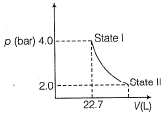Thus, work done is

Solution:

Work done in isothermal process
W= -2.303 nRT log P1/P2
here n=1 and R =8.314  and T= 300 P1=4and P2=2.
W= -1724 J
So option A is correct.

QUESTION: 9

A " 1/4 HP" electric motor uses 187 W of electrical energy while delivering 35 J of work each second. How much energy must be dissipated in the form of friction (heat)?

Solution:

We know that power is energy per second. So by this definition, 187W means 187J/s energy is given.
And 35J work is done.
So energy dissipated in the form of friction= 187 - 35 = 152J

QUESTION: 10

1 mole of a diatomic gas is contained in a piston. It gains 50.0 J of heat and work is done on the surrounding by the system is -100 J. Thus,

Solution:
*Multiple options can be correct
QUESTION: 11

Direction (Q. Nos. 11-15) This section contains 5 multiple choice questions. Each question has four choices (a), (b), (c) and (d), out of which ONE or  MORE THANT ONE  is correct.

Q. The reversible expansion of an ideal gas under adiabatic and isothermal conditions is shown in the following figure. Select correct statement.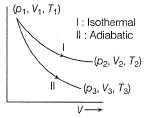[IIT JEE 2012]

Solution:

T1 = T2 because process is isothermal
Work done in adiabatic process is less than in by isothermal process as area under isothermal curve is more than adiabatic curve.
In adiabatic processes, expansion is done by using internal energy. Hence it decreases while in the isothermal process, temperature remains constant. So   there is no change in internal energy.

*Multiple options can be correct
QUESTION: 12

A piston filled with 0.04 mole of an ideal gas expands reversibly from 50.0 mL to 375.0 mL at constant temperature of 37.0°C. As it does, it absorbs 208 J of heat. The value of q and W for the process will be (R = 8.314 J mol-1 K-1, In 7.5 =2.01)

[JEE Main 2013]

Solution: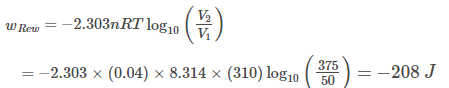*Multiple options can be correct
QUESTION: 13

A sample containing 1.0 mole of an ideal gas is expanded isothermally and reversibly to ten time of its original volume, in two separate experiments. The expansion is carried out 300 K and at 600 K, respectively. Choose the correct option.

Solution:

Work done in isothermal process, w = nRTln(V2/V1)
w600/w300 = 1×R×600×ln(10)/ 1×R×300ln(10) = 2
∆U = 0 for isothermal processes.

*Multiple options can be correct
QUESTION: 14

An ideal gas in a thermally insulated vessel at internal pressure p1 volume V1 and absolute temperature T1 expands irreversibly against zero external pressure, as shown in the diagram. The final internal pressure, volume and absolute tem perature of the gas are p2, V2 and T2, respectively. For this expansion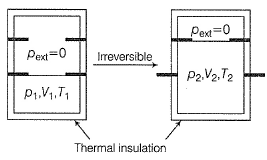Solution:

Since the vessel is thermally insulated, q = 0
Further since,Pext = 0, so w = 0,hence ∆U = 0
Since ∆T = 0, T,2 = T1 and P2V2 = P1V1
However, the process is adiabatic irreversible, so we can’t apply P2V2γ = P1V1γ

*Multiple options can be correct
QUESTION: 15

Based on following figure,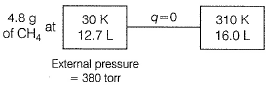Solution:
QUESTION: 16

Direction (Q. Nos. 16-17) This section contains a paragraph, wach describing theory, experiments, data etc. three Questions related to paragraph have been given.Each question have only one correct answer among the four given options (a),(b),(c),(d).

A fixed mass m of a gas is subjected to transformation of states from K to L to M to N and back to K as shown in the figure.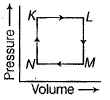Q. The succeeding operations that enable this transformation of states are

Solution:

From K to L; volume increases(sample is expanding) t = heating
From L to M; pressure decreases(molecules are moving far) = cooling
From M to N; volume decreases = cooling
From N to K; pressure increasing = heating

QUESTION: 17

A fixed mass m of a gas is subjected to transformation of states from K to L to M to N and back to K as shown in the figure.Q. A pair of isochoric processes among the transform ation of states is

Solution:

Isochoric process is that process where volume remains constant.
From the graph, we va say that for L→M and for N→K, volume remains constant.

QUESTION: 18

Direction (Q. Nos. 18) Choice the correct combination of elements and column I and coloumn II  are given as option (a), (b), (c) and (d), out of which ONE option is correct.

I. A sample of an ideal gas underwent an expansion against a constant external pressure of 1.0 bar from 1.0 m3, 10.0 bar, and 273 to 10.0 m3, 1.0 bar and 273 K. Work done by the system on the surroundings is W1 (in J).

II. The external pressure was increased to 10.0 bar and the gas sample was isothermally compressed from 10.0 m3 and 1.0 bar to 10 m3 and 10.0 bar.
Work done on the system by the surroundings is W2 (in J).

III. Total work done is W3 (in J).

IV. Least amount of work needed to restore the system to the original p-V conditions is W4 (in J) .

Match W1 W2, W3 and W4 in Column I with corresponding values in Column II.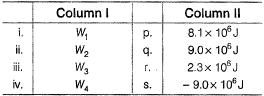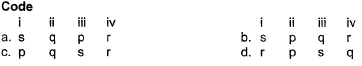Solution:
*Answer can only contain numeric values
QUESTION: 19

Direction (Q. Nos. 19 and 20) This section contains 2 questions. when worked out will result in an integer from 0 to 9 (both inclusive).

Q. About 1 dm3 gastric juice are produced per day in the human body from other body fluids such as blood, whose pH is 7.6021 [H+] = 10-pH] at 53°C. Calculate the minimum work (in kJ) required to produce 1 dm3 gastric juice may be taken as unity.

Solution:

Blood → Gastric Juice
pH = 7.6021
[H+] = 2.5×10-8
∆G° = -RTlnK
= - RTln [1/2.5×10-8]      (since, concentration of gastric juice be taken as unity and we have blood in reactant)
= 47443.26
Now for 1 mole(22.4L) we have Energy = 47443.26
So, for 1dm3(1 L), we have
=47443.26/22.4
=2 kJ
And so according to me, the correct answer is 2 and not 4.

*Answer can only contain numeric values
QUESTION: 20

One mole of an ideal gas, Cp = 4 R at 300 K Is expanded adiabatically to n times the original volume. What is value of n if temperature falls by 150°?

Solution:

Cp - Cv = R
4R - Cv = R
Cv = 3R
γ = Cp/Cv = 4/3
T1/T2 = (v2/v1)(4/3 - 1)
300/150 = (v2/v1)1/3
2 = (v2/v1)1/3
8 = v2/v1
v2 = 8v1
Therefore n = 8